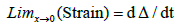Reach Us+32-10-28-02-25
Navier-Stokes Clay Institute Millennium Problem Solution | OMICS International
Journal of Physical Mathematics
All submissions of the EM system will be redirected to Online Manuscript Submission System. Authors are requested to submit articles directly to Online Manuscript Submission System of respective journal.

# Navier-Stokes Clay Institute Millennium Problem Solution

Cusack P*

*Corresponding Author:
Cusack P
Independent Researcher
Tel: (506) 214-3313
E-mail: [email protected]

Received Date: February 08, 2016; Accepted Date: April 29, 2016; Published Date: May 05, 2016

Citation: Cusack P (2016) Navier-Stokes Clay Institute Millennium Problem Solution. J Phys Math 7:176. doi: 10.4172/2090-0902.1000176

Copyright: © 2016 Cusack P. This is an open-access article distributed under the terms of the Creative Commons Attribution License, which permits unrestricted use, distribution, and reproduction in any medium, provided the original author and source are credited.

Visit for more related articles at Journal of Physical Mathematics

#### Abstract

This paper provides the solution to the Navier-Stokes Clay Institute Problem. The Golden Mean parabola is a solution to this equation. The solution shows that the Navier Stokes Equation is smooth.

#### Keywords

Quantum physics; Elementary Particle Theory; Navier-Stokes

#### Introduction

In three space dimensions and time, given an initial velocity field, there exists a vector velocity and a scalar pressure field, which are both smooth and globally defined, that solve the Navier-Stokes equations .

#### Explanation

The Navier Stokes equation:

ρ [du/dt+u*Δ u]=Δ*δ +F

where ρ=density

Du/dt=velocity

U=position

Δδ=Shear

F=all other forces

The solution to this equation is the root of the Golden Mean Equation where the variable is t time explained in Figure 1.

G.M=1.618

First, let’s break down the components as follows.

Density=ρ

ρ=M/Volume

For an ellipsoid with axis 1 × 8 × 22 (or 3 × 24 × 66) has a volume of

19905 and a Surface area of 1 shown in Figure 2.

Mass M=1/c^4

Strain=sigma/E

E=1/0.4233=1/(π)D=E*sigma’=1/0.4233*(P’/A”)

where P is constant

A’=circumference=2π R

Let R=1/2

A=(πR^2)’=2π (R=π)

Delta=1/(0.4233) *P/π

P=(2*s)=(2*4/3)=8/3=2.667

Delta=2.022

Y=e^-t* cos t=dM/dt

2.02=e>^(-t)(-sin t)

Solving for t:

T=114.59°

Substituting:

E^(-2) (sin 2)=1/81=1/c^4

Where “c” is a fourth order tensor and is also the gradient or “Del”.

Plane ax+by+cz=0

Sin θ=c=2.9979293

Sin t=3

T=171°F

Sin θ=0.1411 1/sin θ=M=0.858=Energy=sin 1

E=|s||t|sin θ

θ=60 degrees for Mohr-Coulomb theory illustrated in Figure 3.

E=(1.334) (1) sin 60°=115.5

θ=171°

Sin 171°=0.1411 0.858

Sigma=E strain

If Surface Area=1

F=sigma

F=E strain

0.858=115.5 *strain

Strain=1

Now the Polar Moment of Inertia for the cross section of the ellipsoid is shown in Figure 4:

J=π/2*(c2)^4-π/2*(c1)^4)

J=π/2(13.622)^4-π/2*(2668)

The universe is 13.622 Billion LY across . The Hole in the middle is a=0.2668 Billion LY across.

J=4672

Now the Shear component, is is given by the equation

Tau max=Tc/J

Tau max=(0.4233)(3)/4672 [MECHANICS OF MATERIALS, BEER ET AL]

=2.718

=base e

Referring to the original equation, we now have the density, the mass, the gradient, the shear, and f=0. All that remains is the acceleration, velocity, and position shown in Figure 5.

Delta=PL/AE [ibid]

Delta’=(dP/dt)(dL/dt)/(dA/dt)(dE/dt)

dP/dt=d(sin θ)=-cos θ)

dL/dt=velocity

dA/dt=circumference=2πR

dE/dt=1 (Newtonian Fluid)

delta’=cos theta/(2π (1)* delta’

cos θ=2Pi

Substituting these parameters in to the original equation:

s[(1)-(1/s) *c* (1/s)=Tau max

s^3-sc-e=(4/3)-32.718=1.615~1.618=G.M.

=Ln (1/t)=1.615

where Y=0.2018=e^t cos 1 (dampened cosine curve)

T0-t=1-0.9849=0.015=1/6.66=3/2 (Mass Gap)

E^(3/2)=4.4824=Mass

Ln (1/t)=t

Ln y’=y

So the Navier Stokes is solved by the Golden Mean Parabola 

t=1/(t-1)

t^2-t-1=0,

#### Conclusion

Thus t=Rho[du/dt+u* del u]-Del * sigma -F

where t^2-t-1=0

This parabola is smooth.

The Density=rho/M/Volume is smooth because the Volume of an ellipsoid is smooth. The Mass is smooth because the M=1/c^4. C^4 is smooth.

The Velocity du/dt is a parabola so its derivative is smooth. The position u is a scaler. Its derivative is constant.

Del is the gradient which is c^4. Its derivative is the volume of a sphere equation. It is smooth.

The Shear Tau max is smooth since it is Torque *c/J. Torque is the force=sin theta. Its derivative is smooth. C is a constant. Its derivative is a constant. And the Polar Moment of Inertia π/2(c2-c1)^4. Its derivative is smooth.

The Velocity du/dt is a parabola so its derivative is smooth. The position u is a scaler. Its derivative is constant.

Del is the gradient which is c^4. Its derivative is the volume of a sphere equation. It is smooth.

The Shear Tau max is smooth since it is Torque *c/J. Torque is the force=sin theta. Its derivative is smooth. C is a constant. Its derivative is a constant. And the Polar Moment of Inertia π/2(c2-c1)^4. Its derivative is smooth.

So the Navier Stokes Equation is smooth.

Volume of Sphere=4/3 π (2.9978929)^3=112.8

c=2.997929

Sigma/E=strain

Sigma/F/Surface Area

S.A=1

E=1/0.4233=1/cuz

strain=F/E=2.667/1/0.4233=112.8

This means that the forth order tensor, the speed of light, is as smooth as a sphere. That is why the Navier-stokes Equation is smooth.

#### References

Select your language of interest to view the total content in your interested language

### Article Usage

• Total views: 9538
• [From(publication date):
June-2016 - Nov 15, 2019]
• Breakdown by view type
• HTML page views : 9361Can't read the image? click here to refresh